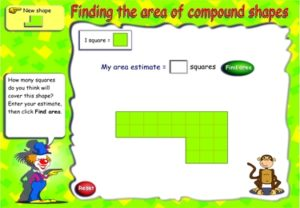## Area of Compound Shapes – ScootleAdd to Bookmarks### Find the area of Compound Shapes

Find the area of compound shapes based on rectangles on a grid.

Explore how the formula works for finding a rectangle’s area.

Information

• Estimate the total area.
• Fill the rectangles with unit blocks
• Calculate the area of each rectangle
• Sum the rectangle areas to find the total area.